Function Repository Resource:

# EllipseProperties

Get an Association of the properties of a given ellipse

Contributed by: Wolfram|Alpha Math Team
 ResourceFunction["EllipseProperties"][expr,{x,y}] returns an Association of properties of the ellipse defined by expr in terms of the variables x and y.

## Details and Options

The input expr can be an equation or an Association of ellipse properties. In this case, ResourceFunction["EllipseProperties"] will calculate and return the full set of properties.
Ellipse properties include "Equation", "Foci", "Vertices", "Covertices", "Eccentricity", "Center", "SemimajorAxisLength", "SemiminorAxisLength", "AreaEnclosed", "SemiLatusRectum", "Directrices", "AxesOfSymmetry", "Circumference", "RotationAngle" and "BaseConic".
The "BaseConic" property is an Association of the properties "Equation", "RotationAngle" and "DisplacementFromOrigin", where "Equation" represents an isomorphic conic section based at the origin and aligned with the x-axis.
If the given expr describes a conic section other than a ellipse, ResourceFunction["EllipseProperties"] will issue a message and return an Association of properties pertaining to the correct conic section.

## Examples

### Basic Examples (1)

Compute the properties of an ellipse:

 In:=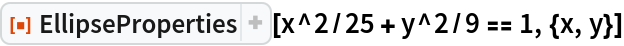Out=### Scope (3)

Compute the properties of a ellipse described using other properties:

 In:=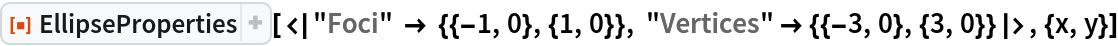Out=Compute the properties of a skew ellipse (not aligned with the x- or y-axis) described by some properties:

 In:=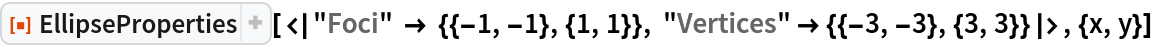Out=In:=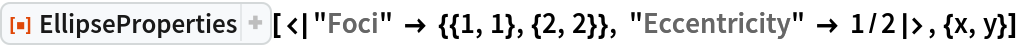Out=Compute the properties of a circle as a special case of an ellipse:

 In:=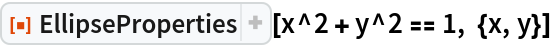Out=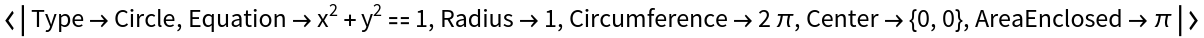In:=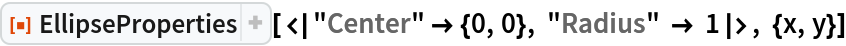Out=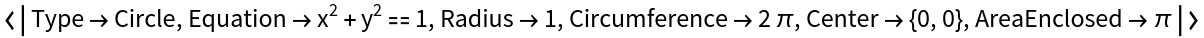### Applications (2)

Compute the properties of a general ellipse centered at {h,k} with radius semi-axis lengths a and b:

 In:=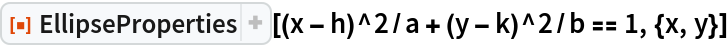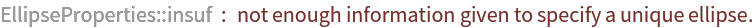Out=Note that we have to give a constraint on a and b in order to fully define the ellipse:

 In:=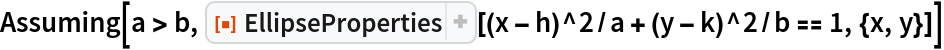Out=### Properties and Relations (1)

Use the resource function InteractiveConicPlot to visualize the results of EllipseProperties:

 In:=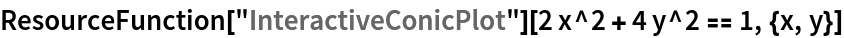Out=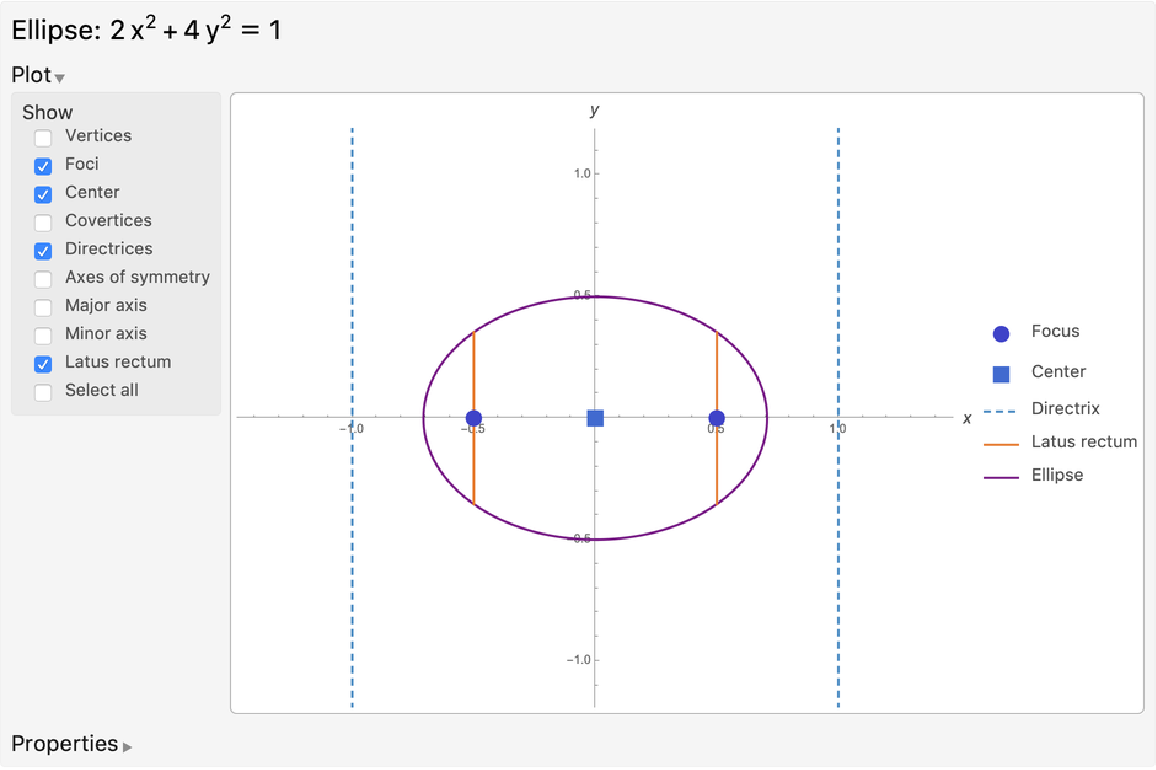### Possible Issues (1)

If the input equation or Association does not describe an ellipse but describes another conic section, EllipseProperties will return an Association of properties pertaining to the correct conic section, including its "Type" ("Parabola", "Hyperbola" or "Circle"):

 In:=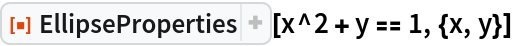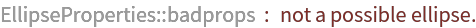Out=## Publisher

Wolfram|Alpha Math Team

## Version History

• 2.0.0 – 23 March 2023
• 1.0.0 – 01 May 2020